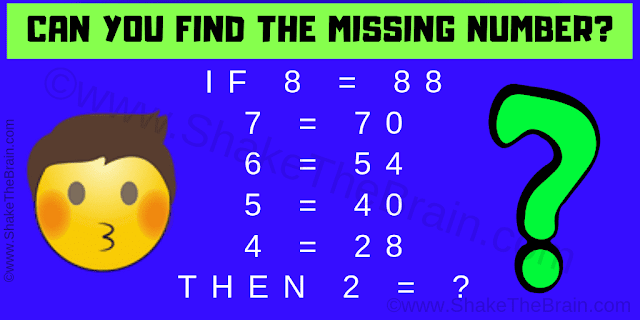## Friday, July 19, 2019

It is Logical Math Puzzle to challenge your brain. In this Logic math puzzle, your challenge is to find the value of the missing number which will replace the question mark. Find the logical relationship among the given numbers and decipher the logic used in these logical equations. Once you are able to decode the logic, find the value of the missing number.Can you solve this Logical Math Puzzle?
Answer of this "Logical Math Puzzle", can be viewed by clicking on the answer button.

1.2.